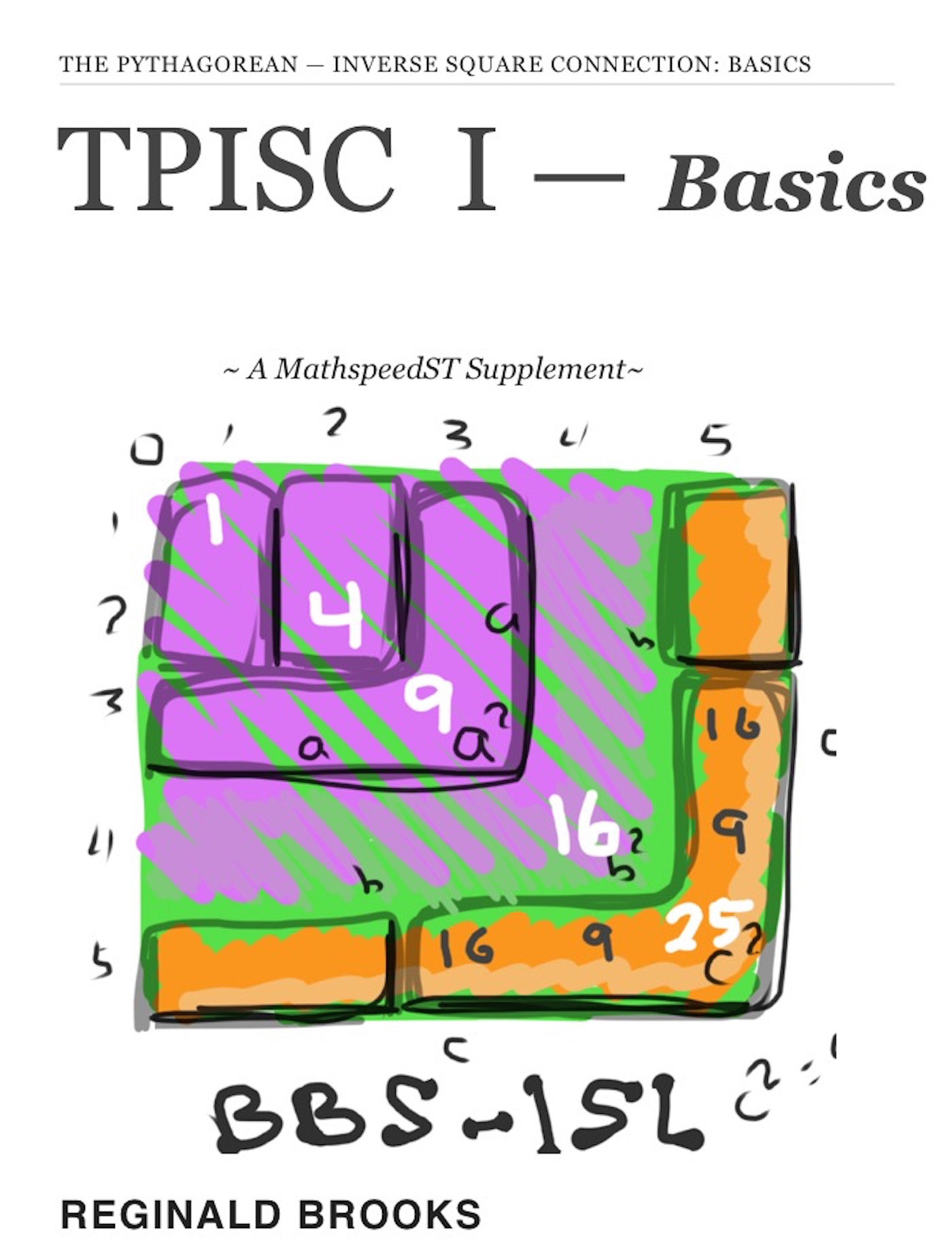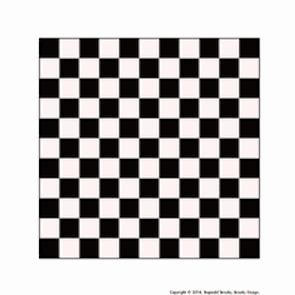# TPISC I: Basics: The Pythagorean — Inverse Square Connection ~A MathspeedST Supplement~Statement

Introducing TPISC I: Basics, an ebook. TPISC is a supplement to MathspeedST (published in November, 2013).

While LightspeedST addressed the ultimate question: “Why is the speed of light constant?”MathspeedST addressed the fundamental, underlying question: “How can information be ubiquitous, effectively traveling FASTER than the speed of light?”

TPISC describes the Pythagorean Triangles (Triples) and their ubiquitous connection to the unfolding Inverse Square Law (ISL) as depicted in the BBS-ISL Matrix.

The BBS-ISL Matrix (Brooks Base Square Matrix) presents the ISL as a natural, whole integer number matrix grid that expands to infnity.

TPISC is also, by definition, grounded in natural, whole integer numbers forming the sides of the Pythagorean Triples (PT). The PTs also expand to infinity and are deeply embedded within the BBS-ISL Matrix throughout their co-expansion. Enter TPISC.

TPISC I: Basics provides the simple, introductory geometrical (Visual) and algebraic (Calculation) mathematical basis for the accounting and distribution of all Pythagorean Triple Triangles — primitive and non-primitive — directly on the BBS-ISL Matrix.

This freely available interactive ebook brings together under one publication the intersection of two geometries: The Pythagorean Triangles and the BBS-ISL Matrix under the title TPISC I: Basics. It includes hundreds of images, a number of videos and lots of test-yourself, interactive widgets in presenting the layout, proofs, and patterns of the Pythagorean Triples and their ISL-derivation and, ultimately, the mathematical basis of their connection with the matrix. Ages 13 and up, or younger if you are up to the task. Basically, if you can add and subtract, meaning you can multiply and divide, you can easily gain access to TPISC. Both TPISC and the BBS-ISL Matrix are composed solely of natural, whole integer numbers. No decimals, fractions, integrals, differentials,.... Nothing you can't handle! But plenty in the Advanced form to keep you going for...(ever!).

A new paradigm in the visualization of basic mathematics is presented!

Description:

TPISC I: Basics TPISC I: The Pythagorean - Inverse Square Connection: Basics reveals the presence, proofs and and overall distribution of the Pythagorean Triples (PTs) upon the BBS-ISL Matrix. The distribution was shown as occurring on, and only on, each and every Row/Column that contained AREA Squares. Scan the Rows and if they contained Pair-Sets of these AREA Squares you would always find a PT there.

~~~

Abstract

There is a simple whole number (integer) matrix grid table that every possible whole number Pythagorean Triangle — a.k.a. Pythagorean Triple — can be found and proved. The Brooks Base Square - Inverse Square Law (BBS-ISL) Matrix is an infinitely expandable grid that reveals ALL Pythagorean Triples — both Primitive Triples (PPT) and their non-Primitive multiples (nPTT). An extremely simple geometric AREA proof of the Pythagorean Theorem — c2=a2+b2 — is built into the BBS-ISL Matrix. Not only does the Inverse Square Law describe, define and quantify our most important energies (and their expressions as force) — gravity, light, sound, electromagnetism… — the BBS-ISL Matrix grid is composed of Pythagorean Triples crisscrossing over much of the entire grid. An intimate inter-connection way beyond a simple, casual association has been revealed. The Dickson Method confirms, validates and provides insight into the generation of ALL Pythagorean Triples.

~~~

Introduction

The reason that this work is being presented is precisely because the very nature of that geometric relationship of the Pythagorean Triangle (PT) — whereby the AREA of the square of the long side (hypotenuse) is equal to the sum of the Squares of the two shorter SIDES (legs) — is so intimately related to the Inverse Square Law (ISL), as depicted in the BBS-ISL Matrix, that a cause and effect relationship between the two is unavoidable.

That the BBS-ISL Matrix provides the simplest, most intuitively obvious proof to the Theorem directly on the grid only supports the argument. Every possible PT, and its proof, is visually and mathematical present. The Dickson Method confirms this.

~~~

Conclusion

More than adequately covered in the literature, writings, songs and art of all cultures, it has been an attempt here to present an entirely new manner of looking at the Pythagorean Triples, their proofs, distribution and ultimately their inter-connections. As it turns out, the inter-connections with the BBS-ISL Matrix are what has become the main theme. As the Inverse Square Law (ISL) upon which the BBS-ISL Matrix is formed is, in many respects, one of, if not the main, “laws” or relationship informing principles in all of science — most especially physics and mathematics. That the PT should be so intimately born, described and proved within that matrix is not without great introspection. If the ISL is fundamentally about how influence is distributed — spread out and diluted — over SpaceTime (ST), then the Pythagorean Theorem is a natural, built-in measure of that.

~~~

1. Introduction 2. TPISC—BBS-ISL (Visualizations) 3. Dickson Method (Calculations) 4. Inter-Connections 5. TPISC II: Advanced (preview 1) 6. Summary 7. Conclusion 8. Links & References 9. Appendix 10. Next—TPISC Advanced (preview 2) 11. Review 12. Next: BBS - ADVANCED (preview) 13.

~~~

TPISC II: The Pythagorean - Inverse Square Connection: Advanced — a separate ebook — profoundly enlarges upon the Dickson Method — giving the Extended Dickson Method — which has opened the doors to the real connection between the ISL and the PTs. Patterns of Fractal-like Relationships, is a descriptive phrase loaded with the implications that the PTs, while ubiquitously embedded throughout the infinite BBS-ISL Matrix, grow within it in a profoundly fractal-like pattern. Parsing these into r-set and s-set groups becomes key in determining the pattern distribution of ALL PTs.

~~~

Platforms

This is a multi-touch, interactive ebook made with iBooks Author and published in the Apple iBooks Store. Currently, it requires an iOS or MAC device and the free iBooks app, to download and read..

Multi-touch, interactive eBook: TPISC_I: Basics ebook

Selected SCREENSHOTS for TPISC I: Basics

Artist Link in iTunes iBooks Store: Reginald Brooks~---

~ MathspeedST: The Ubiquitous Information of Numbers white paper
~ LightspeedST: Leapfrogging @ The Speed of Light
T H E   U B I Q U I T O U S   I N F O R M A T I O N    O F    N U M B E R S new media net.art project
~ MathspeedST: Leapfrogging Lightspeed FASTER than The Speed of Light MOVIES
The contents of TPISC I: Basics, and both MathspeedST and LightspeedST, can be viewed as White Papers in the Art Theory 101 links below.

Relevant White Papers by the Author

http://www.brooksdesign-ps.net/Reginald_Brooks/Code/Html/arthry5.htm

titles and topics:

### LightspeedST: The Complete New Media net.art project (HOME)

KEYWORDS TAGS:
TPISC, The Pythagorean - Inverse Square Connections, Pythagorean Triangles, Pythagorean Triples, primitive Pythagorean Triples, non-primitive Pythagorean Triples, Pythagorean Theorem, Pythagorus Theorem, The Dickson Method, BBS-ISL Matrix, Expanded Dickson Method, r-sets, s-set, t-sets, Pair-sets, geometric proofs, MathspeedST, leapfrogging LightspeedST FASTER than the speed of light, Brooks (Base) Square- Inverse Square Law (ISL), BBS-ISL matrix grid, The Architecture Of SpaceTime (TAOST), The Conspicuous Absence Of Primes (TCAOP), A Fresh Piece Of Pi(e), AFPOP, Numbers of Inevitability,LightspeedST, Teachers, Educators and Students (TES), number theory, ubiquitous information, FASTER than the speed of light, primes, prime numbers, fractals, mathematics, Universe, cosmos, patterns in number.

Art Theory 101 / White Papers Index
 PIN: Pattern in Number...from primes to DNA. | PIN: Butterfly Primes...let the beauty seep in. | PIN: Butterfly Prime Directive...metamorphosis. | PIN: Butterfly Prime Determinant Number Array (DNA) ~conspicuous abstinence~. | GoDNA: the Geometry of DNA (axial view) revealed. | SCoDNA: the Structure and Chemistry of DNA (axial view). | The LUFE Matrix | The LUFE Matrix Supplement | The LUFE Matrix: Infinite Dimensions | The LUFE Matrix: E=mc2 | Dark Matter=Dark Energy | The History of the Universe in Scalar Graphics | The History of the Universe_update: The Big Void | Quantum Gravity ...by the book | The Conservation of SpaceTime | LUFE: The Layman's Unified Field Expose` | GoMAS: The Geometry of Music, Art and Structure ...linking science, art and esthetics. Part I | Brooks (Base) Square (BBS): The Architecture of Space-Time (TAOST) and The Conspicuous Absence of Primes (TCAOP) - a brief introduction to the series net.art index | netart01: RealSurReal...aClone, 2001 | netart02: Hey!Ufunk'n with my DNA? | netart03: 9-11_remembered | netart04: Naughty Physics (a.k.a. The LUFE Matrix) | netart05: Your sFace or Mine? | netart06: Butterfly Primes | netart07: Music-Color-ISL | netart08: BBS-ISL matrix | netart09: BBS-interactive (I) | netart10: Sunspots and Solar Flares | netart11: Music-Color-ISL (II-III) | Art Theory 101: PIN, DNA, LUFE Matrix, GoMAS, BBS index | home Copyright 2015-19, Reginald Brooks, Brooks Design. All rights reserved. iTunes, iTunes Store, iBooks, iBooks Store, iBooks Author, Mac OS are registered® trademarks of Apple Inc. and their use on this webpage does not reflect endorsement by Apple Inc.